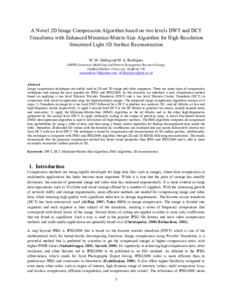# A novel 2D image compression algorithm based on two levels DWT and DCT transforms with enhanced minimize-matrix-size algorithm for high resolution structured light 3D surface reconstruction

SIDDEQ, M and RODRIGUES, Marcos (2015). A novel 2D image compression algorithm based on two levels DWT and DCT transforms with enhanced minimize-matrix-size algorithm for high resolution structured light 3D surface reconstruction. 3D Research, 6 (3), p. 26.Preview
PDF
root.pdf - Accepted Version
Creative Commons Public Domain Dedication.

## Abstract

Image compression techniques are widely used in 2D and 3D image and video sequences. There are many types of compression techniques and among the most popular are JPEG and JPEG2000. In this research, we introduce a new compression method based on applying a two level Discrete Wavelet Transform (DWT) and a two level Discrete Cosine Transform (DCT) in connection with novel compression steps for high-resolution images. The proposed image compression algorithm consists of 4 steps: 1) Transform an image by a two level DWT followed by a DCT to produce two matrices: DC- and AC-Matrix, or low and high frequency matrix respectively; 2) apply a second level DCT to the DC-Matrix to generate two arrays, namely nonzero-array and zero-array; 3) apply the Minimize-Matrix-Size (MMS) algorithm to the AC-Matrix and to the other high-frequencies generated by the second level DWT; 4) apply arithmetic coding to the output of previous steps. A novel Fast-Match-Search (FMS) decompression algorithm is used to reconstruct all high-frequency matrices. The FMS-algorithm computes all compressed data probabilities by using a table of data, and then using a binary search algorithm for finding decompressed data inside the table. Thereafter, all decoded DC-values with the decoded AC-coefficients are combined into one matrix followed by inverse two level DCT with two level DWT. The technique is tested by compression and reconstruction of 3D surface patches. Additionally, this technique is compared with JPEG and JPEG2000 algorithm through 2D and 3D RMSE following reconstruction. The results demonstrate that the proposed compression method has better visual properties than JPEG and JPEG2000 and is able to more accurately reconstruct surface patches in 3D.

Item Type: Article Cultural Communication and Computing Research Institute > Communication and Computing Research Centre https://doi.org/10.1007/s13319-015-0055-6 p. 26 Marcos Rodrigues 02 Jun 2015 09:02 18 Mar 2021 04:46 https://shura.shu.ac.uk/id/eprint/10049View Item